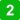# Digital work orders Automotive Electrics Trainer

Practical assignments with Basic electrical Trainer (basic), 11 h 11 minThis practical gives you more insight into the various circuits that can occur in electrical engineering. Step by step you will build the various circuits. Then you will perform measurements in order to gain insight into voltages and currents.

Basic electrical Trainer (basic), 21 h 08 min.
After going through the practical assignments you will be able to: Connect various electric circuits; Identify components of an electric circuit; Explain the operation of various electric circuits; Recognise various electric symbols; Perform measurements on various electric circuits.

Preliminary theory with Basic electrical Trainer (basic), 9 h 57 min*
• Electrical fundamentals - basics
• Capacitor - specialist
• Voltmeter - basics
• Ampmeter - basics
• Multimeter autorange - basics
• Kirchhoff's first law - advanced
• Series circuits - basics
• Parallel circuits - basics
Practical assignments with Basic electrical Trainer (basic), 11 h 11 min
• Basic electrical Trainer: Introduction - basics
• Basic electrical Trainer: Connect in series - basics
• Basic electrical Trainer: Connect parallel circuit - basics
• Basic electrical Trainer: Connecting combination circuit - basics
• Basic electrical Trainer: Basic measurements - basics
• Basic electrical Trainer: Measuring voltage - basics
• Basic electrical Trainer: Measuring current - basics
• Basic electrical Trainer: Measuring resistance - basics
• Basic electrical Trainer: Circuit power - basics
• Basic electrical Trainer: Circuit with resistors - basics
• Basic electrical Trainer: Circuit with lamp - basics
• Basic electrical Trainer: Circuit with relay - basics
• Basic electrical Trainer: Circuit with diode - basics

License: You purchase a license for any number of students and teachers with a period of validity of 10 years. This can be extended individually after. The software will be activated on your Electude e-learning domain.

* Separate license may apply for the Preliminary theory.

 Manufacturer: Electude Category: Digital work orders Item number: 12020112 EQF-Level‍:E-Learning‍: